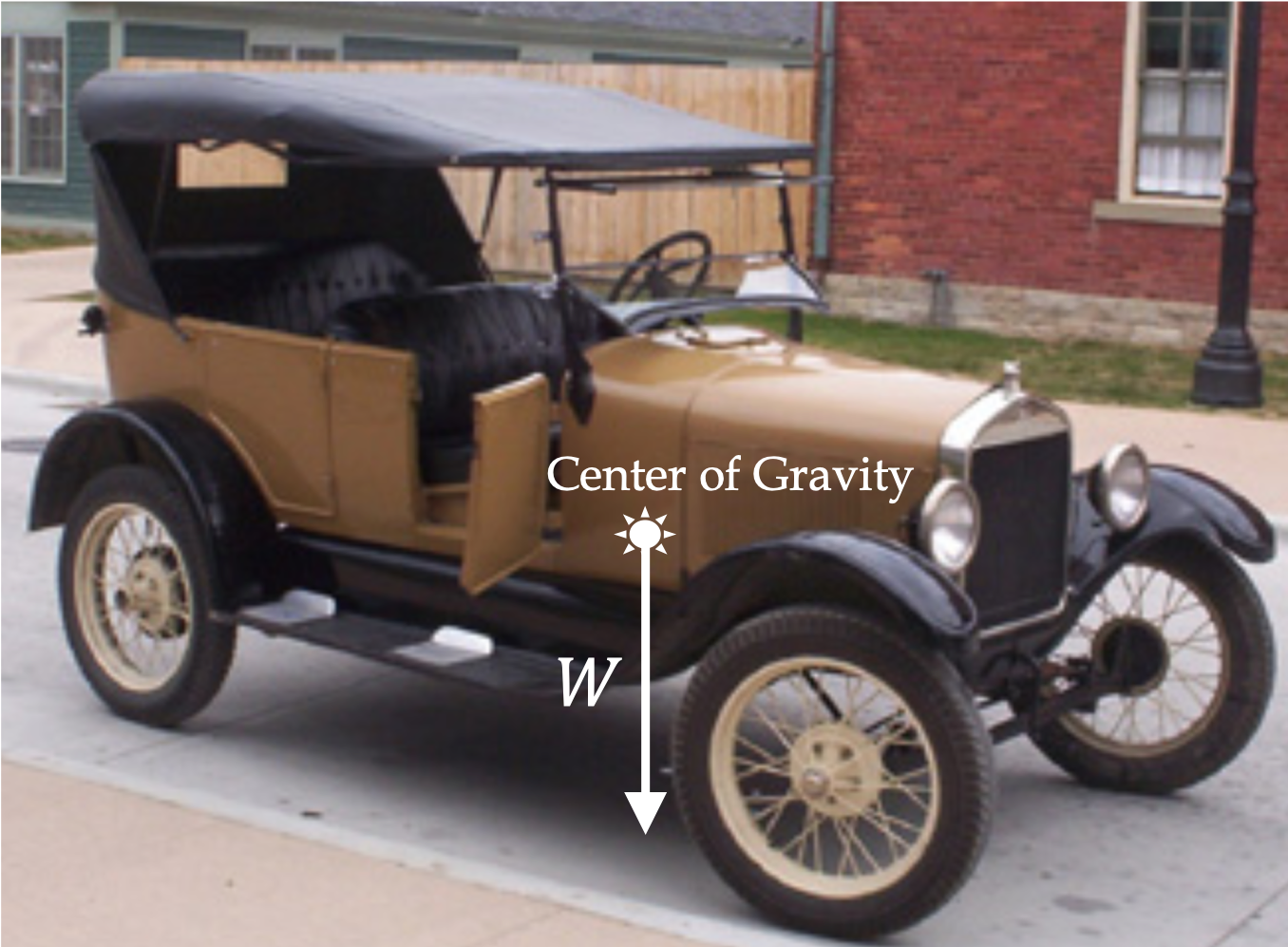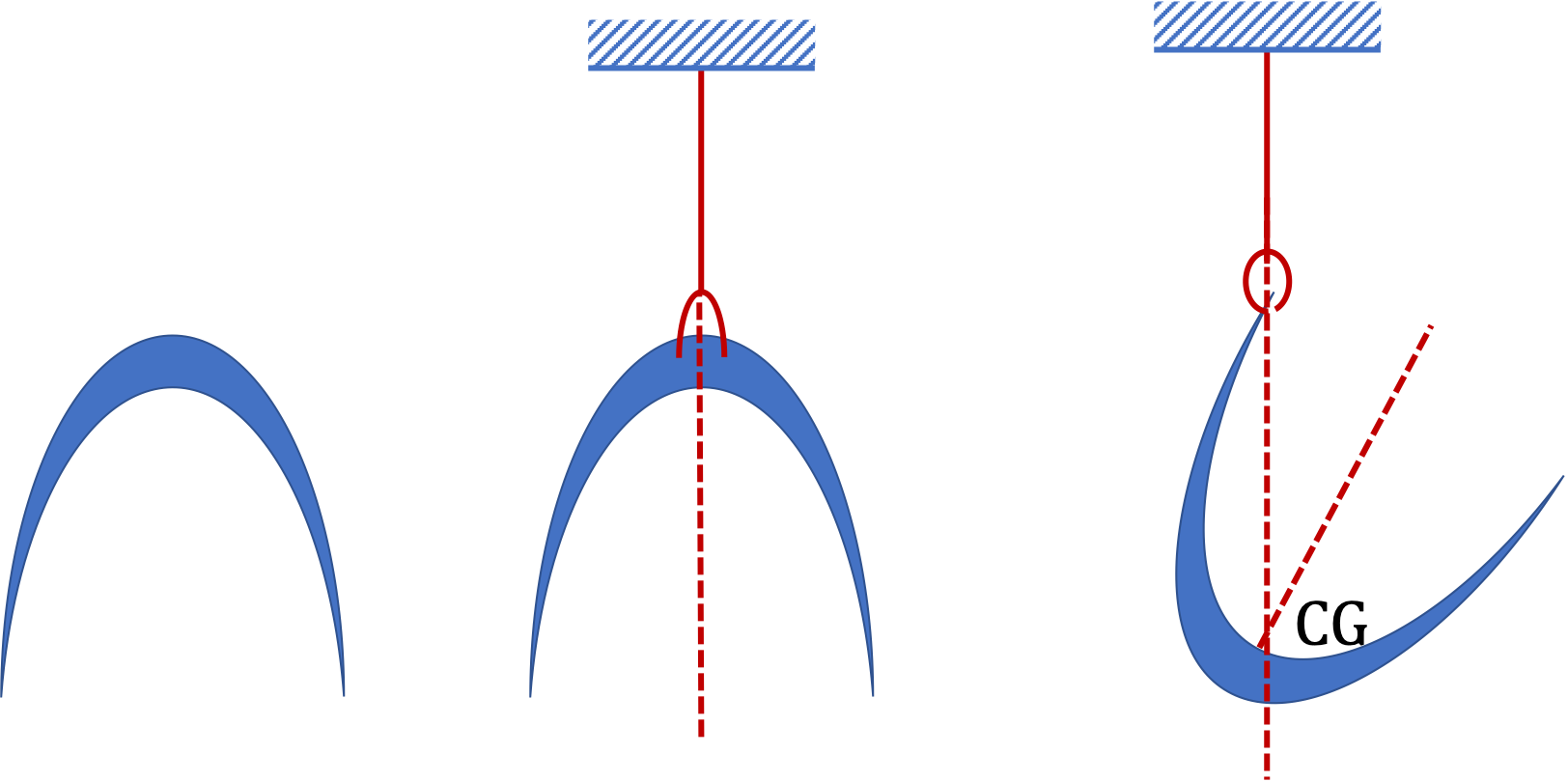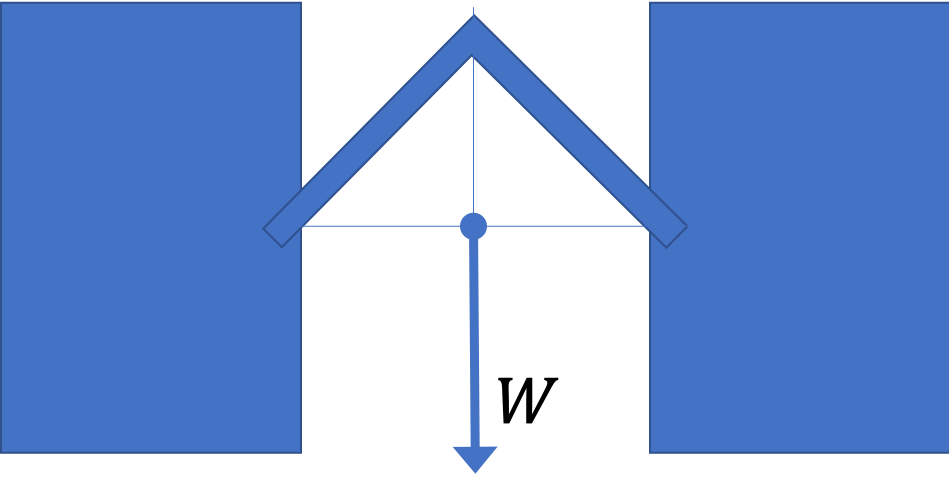## Section6.7Weight

Weight is the name of the force between Earth and a body under study. When weight acts on an object, we simply call it the weight of the object. When the force of weight between you and Earth acts on Earth, it does not make much difference since the main force on Earth is by the Sun, which is a very large force.

We will denote weight of a body by symbol $\vec W\text{.}$

\begin{equation*} \vec W_\text{on object}^\text{by Earth} \text{ denoted by } \vec W. \end{equation*}

Sometimes, we will place a subscript letter to denote the weight on a particular body if there is more than one body of interest in a situation. The magnitude will be the same symbol without the arrow over, $W \text{.}$

The magnitude of weight of an object near Earth, is very nearly independent of the object's distance from Earth. If mass of the object is $m \text{,}$ then magnitude of weight is

\begin{equation} W= m\, g,\label{eq-weight-magnitude}\tag{6.7.1} \end{equation}

where $g$ is called acceleration due to gravity, which has a standard value $9.81 \text{ m/s}^2\text{.}$

The weight acting on an object has a direction pointed vertically down and perpendicular to the locally flat surface of the Earth. This is, of course, a simplifying assumption since gravitational force by Earth will be towards the center of Earth.

Note that although weight is proportional to mass, weight is not mass. Weight is a force, which is a vector, while mass is a simple positive scalar quantity. Also, since $g$ has different values on different heavenly bodies, your weight will different on different planets and moons. For instance, even though your mass will be same, you would weigh approximately one-sixth on the Moon as you weigh on Earth and about $2.5$ times on Jupiter.

We might define mass by the $m = W/g$ formula, i.e., we might say, mass is a physical property of an object that has the value equal to its weight divided by a standard number $g \text{.}$ A spring scale uses this definition of mass.

Center of gravity:

The force of weight on a a particle-like object acts just on the particle. But most regularly encountered bodies, such as car, are made of many particles or parts. Then, it can be shown that the influence of weight on the motion of such a body is same as if weight acted on a special point in space, called the center of gravity.You can find center of gravity by hanging a body by a string. The center of gravity will fall on the line of the string. Then, you would hang the object with string tied at another point. The lines will cross at the center of gravity. This is illustrated in Figure 6.7.2 for a crescent-shaped object.Figure 6.7.2. One can determine center of gravity (CG) of arbitrary shape object by hanging the body by different points and finding the crossing point of hanging lines.

The object shown in the figure has two large masses, each of $200\text{ kg}\text{,}$ with a relatively light bent bar, whose mass may be ignored.(a) What is the weight of the dumb-bell?

(b) What does the weight of the dumb-bell act? Show by drawing an arrow with the tail at the point you think it acts.

Hint

Use definition

(a) $3924\text{ N} \text{,}$ (b) See solution.